• 基于FPGA低通滤波器FIR的设计1 滤波器的特征参数介绍图1 低通滤波器特征参数 如图1所示，低通滤波器的通带截止频率为ωp ,通带容限为α1，阻带截止频率为ωs,阻带容限为α2。通带定义为|ω|≤ωp ,过渡带定义为ωp2 ...


基于FPGA低通滤波器FIR的设计1 滤波器的特征参数介绍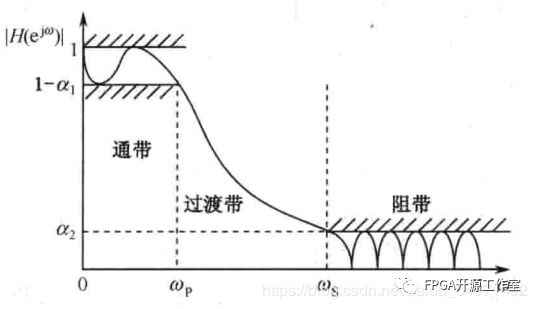图1 低通滤波器特征参数       如图1所示，低通滤波器的通带截止频率为ωp ,通带容限为α1，阻带截止频率为ωs,阻带容限为α2。通带定义为|ω|≤ωp ,过渡带定义为ωp2 设计目标      要求：使用fir滤波器设计一个滤波器系数为15阶的低通滤波器，通带截止频率为1KHZ，采样频率为44.1khz。3 matlab的设计验证close allclear allclc%设计一个低通滤波器 采样频率为44.1khz, 截止频率为1khzFS = 44100; %HZfc = 1000;N  = 15;Q  = 16;%以采样频率的一半，对频率进行归一化处理wn_lpf=fc*2/FS;%采用fir1函数设计FIR滤波器b_lpf=fir1(N-1,wn_lpf);%滤波系数进行量化b_16=round(b_lpf/max(abs(b_lpf))*(2^(Q-1)-1));%求滤波器的幅频响应m_lpf=20*log(abs(fft(b_lpf)))/log(10);b16_lpf=20*log(abs(fft(b_16)))/log(10);%设置幅频响应的横从标单位为Hzx_f=[0:(FS/length(m_lpf)):FS/2];  %绘制单位脉冲响应subplot(221);stem(b_lpf);xlabel('n');ylabel('h(n)');title('低通滤波器的单位脉冲响应','fontsize',8);subplot(222);stem(b_16);xlabel('n');ylabel('h(n)');title('低通滤波器的单位脉冲响应','fontsize',8);%绘制幅频响应曲线subplot(223);plot(x_f,m_lpf(1:length(x_f)));xlabel('频率(Hz)','fontsize',8);ylabel('幅度(dB)','fontsize',8);title('低通滤波器的幅频响应','fontsize',8);subplot(224);plot(x_f,b16_lpf(1:length(x_f)));xlabel('频率(Hz)','fontsize',8);ylabel('幅度(dB)','fontsize',8);title('低通滤波器的幅频响应','fontsize',8);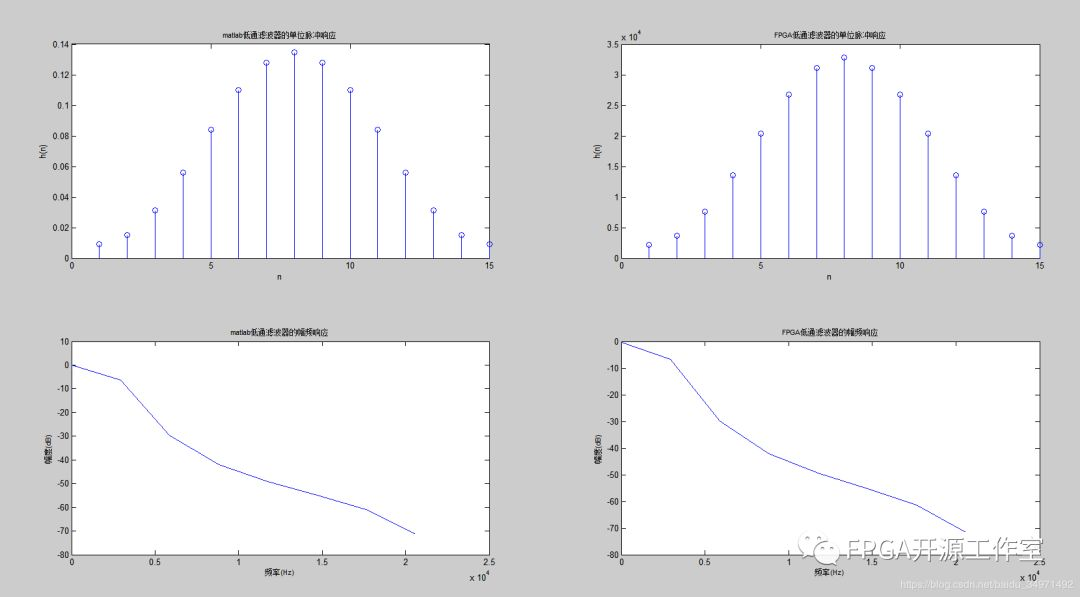图2 低通滤波器的matlab单位脉冲响应和幅频响应4 FPGA信号发生器为了验证低通滤波器，我们设计了测试验证的sin信号发生器。wire [17:0] kfreq= (k<2048)?18’h00333:18’h00FFF;//1khz-5khz产生1khz和5khz的sin波。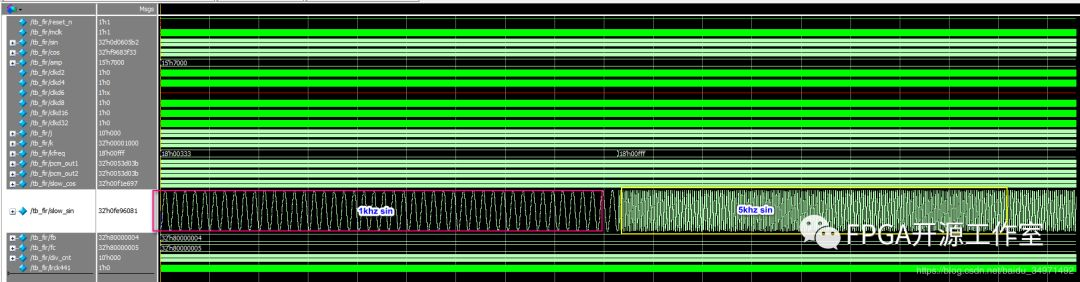图3 信号发生器产生1khz和5khz的sin测试源测试源的FFT分析：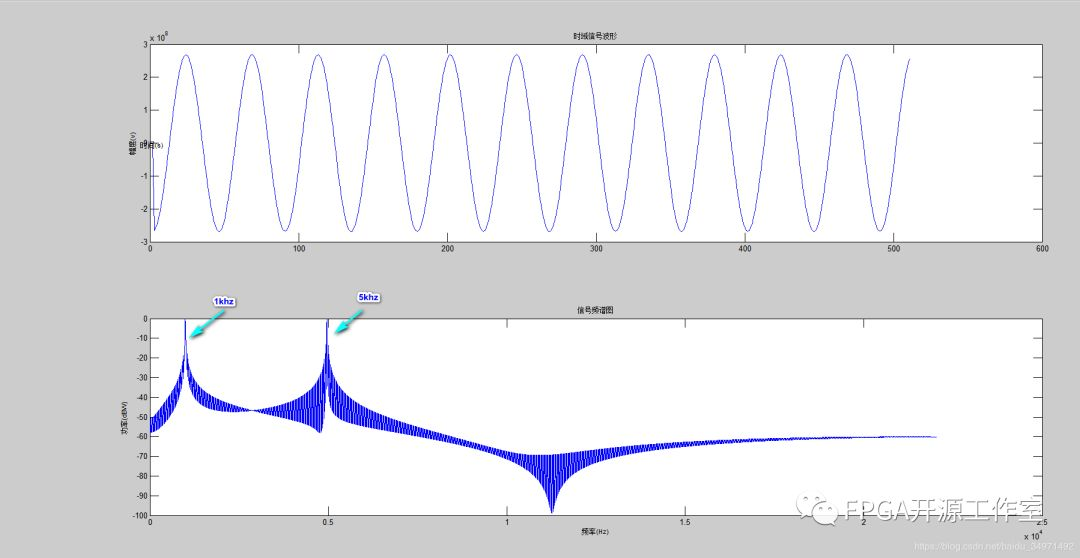图4 测试源数据的matlab分析      由图4的频域图可知，测试源产生了1khz和5khz的sin。5 FPGA的fir设计      Fir滤波器verilog代码设计采取全并行模式。timescale 1ps/1psmodule fir1(           input mclk,//45.1584MHZ	   input reset_n,	   input signed[31:0] pcm_in,	   output signed[31:0] pcm_out);localparam LAST_CYCLE = 1023;reg [9:0] i;reg signed [31:0] pcm1,pcm2,pcm3,pcm4,pcm5,pcm6,pcm7,pcm8,pcm9,pcm10,pcm11,pcm12,pcm13,pcm14,pcm15;reg signed [47:0] pcm1_out,pcm2_out,pcm3_out,pcm4_out,pcm5_out,pcm6_out,pcm7_out,pcm8_out,pcm9_out,pcm10_out,pcm11_out,pcm12_out,pcm13_out,pcm14_out,pcm15_out;reg signed [51:0] pcm_r;wire signed [15:0] coeff1,coeff2,coeff3,coeff4,coeff5,coeff6,coeff7,coeff8,coeff9,coeff10,coeff11,coeff12,coeff13,coeff14,coeff15;assign coeff1 = 2208;assign coeff2 = 3631;assign coeff3 = 7612;assign coeff4 = 13576;assign coeff5 = 20413;assign coeff6 = 26727;assign coeff7 = 31169;assign coeff8 = 32767;assign coeff9 = 31169;assign coeff10 = 26727;assign coeff11 = 20413;assign coeff12 = 13576;assign coeff13 = 7612;assign coeff14 = 3631;assign coeff15 = 2208;assign pcm_out = pcm_r[51:20];always @(posedge mclk or negedge reset_n) beginif(!reset_n) begin    i<= 0;	pcm1<=0;	pcm2<=0;	pcm3<=0;	pcm4<=0;	pcm5<=0;	pcm6<=0;	pcm7<=0;	pcm8<=0;	pcm9<=0;	pcm10<=0;	pcm11<=0;	pcm12<=0;	pcm13<=0;	pcm14<=0;	pcm15<=0;    pcm1_out<=0;	pcm2_out<=0;	pcm3_out<=0;	pcm4_out<=0;	pcm5_out<=0;	pcm6_out<=0;	pcm7_out<=0;	pcm8_out<=0;	pcm9_out<=0;	pcm10_out<=0;	pcm11_out<=0;	pcm12_out<=0;	pcm13_out<=0;	pcm14_out<=0;	pcm15_out<=0;	pcm_r<= 0;  endelse begin    i <= i + 1;if(i == 0) begin	  pcm1<=pcm_in;	  pcm2<=pcm1;	  pcm3<=pcm2;	  pcm4<=pcm3;	  pcm5<=pcm4;	  pcm6<=pcm5;	  pcm7<=pcm6;	  pcm8<=pcm7;	  pcm9<=pcm8;	  pcm10<=pcm9;	  pcm11<=pcm10;	  pcm12<=pcm11;	  pcm13<=pcm12;	  pcm14<=pcm13;	  pcm15<=pcm14;	endif(i==1) begin    	pcm1_out <= pcm1*coeff1;	    pcm2_out <= pcm2*coeff2;	    pcm3_out <= pcm3*coeff3;	    pcm4_out <= pcm4*coeff4;	    pcm5_out <= pcm5*coeff5;	    pcm6_out <= pcm6*coeff6;	    pcm7_out <= pcm7*coeff7;	    pcm8_out <= pcm8*coeff8;	    pcm9_out <= pcm9*coeff9;	    pcm10_out <= pcm10*coeff10;	    pcm11_out <= pcm11*coeff11;	    pcm12_out <= pcm12*coeff12;	    pcm13_out <= pcm13*coeff13;	    pcm14_out <= pcm14*coeff14;	    pcm15_out <= pcm15*coeff15;	endif(i==2) pcm_r<= pcm1_out +pcm2_out+pcm3_out+pcm4_out+pcm5_out+pcm6_out+pcm7_out+pcm8_out+pcm9_out+pcm10_out+pcm11_out+pcm12_out+pcm13_out+pcm14_out+pcm15_out;  endendendmodule     fpga实验modelsim仿真结果，从图5来看1khz几乎保持不变，而5khz波形幅度上被抑制了很多。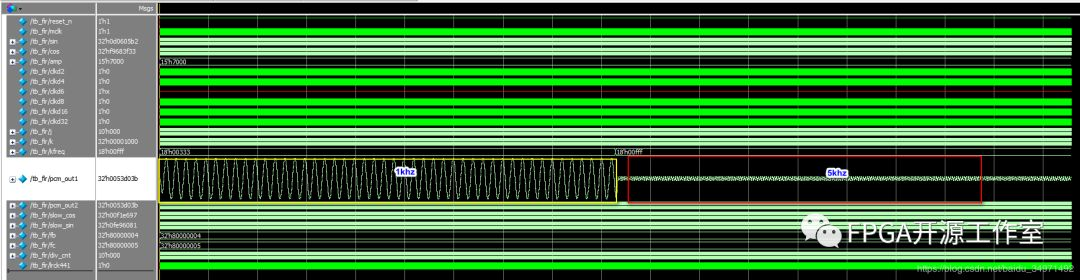图5 modelsim fir实验结果时域波形Matlab fft分析：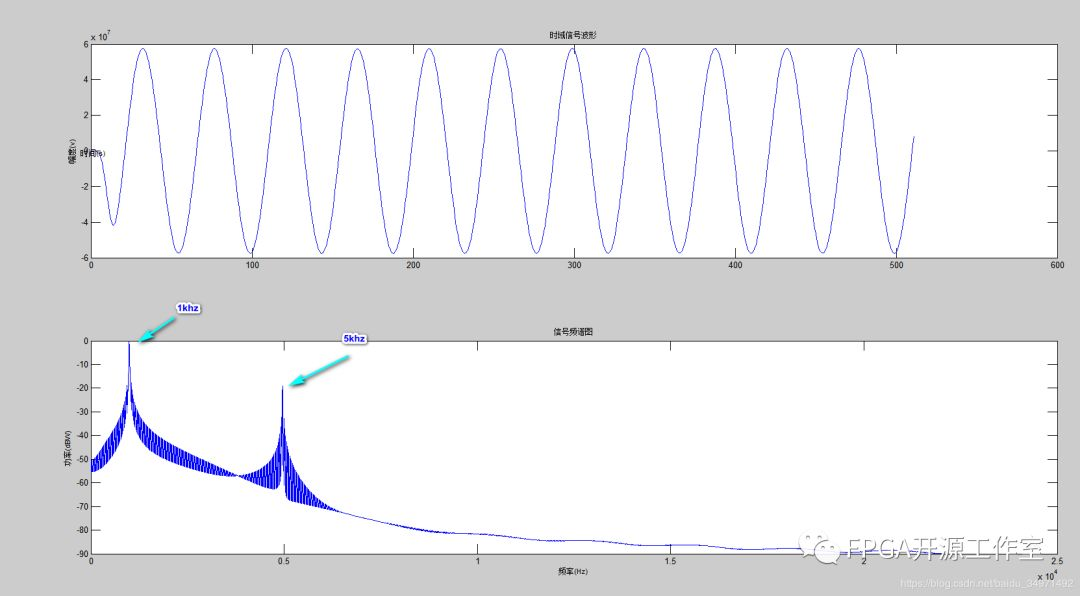图6 matlab分析实验结果      由图2和图6对比，5khz经过滤波后的功率减小了20DB，图2与图5基本一致，fir的低通滤波器全并行设计成功。DSP往期音频总线I2S协议信号的产生信号的基本概念基于LUT的DDS的设计更多精彩推荐，请关注我们万水千山总是情，点个 “好看” 行不行！！！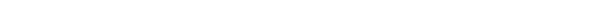展开全文• FIR设计-基于MatlabFIR低通滤波器的设计与仿真.pdf 基于MatlabFIR低通滤波器的设计与仿真.pdf FIR设计 FIR滤波器设计与仿真。
• Matlab 实现振动信号低通滤波 附件 txt 中的数字是一个实测振动信号采样频率为 5000Hz试设计一个长度 为 M=32 的FIR 低通滤波器截止频率为 600Hz用此滤波器对此信号进行滤波 要求 1 计算数字截止频率 2 给出滤波器...
• matlab上实现FIR低通滤波器，并用它实现对噪声的滤除，每一步都加有注释，方便参考，程序运行无误
• 使用MATLAB设计FIR低通滤波器 ** 关于现代通信原理作业。 https://blog.csdn.net/tanghonghanhaoli/article/details/100533581 这是老师给出的提示，里面有比较详细的讲解，下面写一些我的想法。 1. 为什么要设计低...
**
使用MATLAB设计FIR低通滤波器
**
关于现代通信原理作业。
https://blog.csdn.net/tanghonghanhaoli/article/details/100533581
这是老师给出的提示，里面有比较详细的讲解，下面写一些我的想法。
1. 为什么要设计低通滤波器
我们可以看到例子中给的m(t)在时域中已经是物理可实现的信号了，但是在频域上看这个信号的频谱是无限宽的，显然这用计算机是难以处理的，加上Sa信号旁瓣的影响并不是很大，所以我们考虑滤除旁瓣，即使用低通滤波器。
2. 关于如何设计低通滤波器的理论分析
对于例题中是想保留Sa信号±10Hz内的频率分量，即需要设计一个频率范围在±10Hz的低通滤波器h(t)，也就是频域为一个方波信号H(f)。由信号与系统的知识可得，频域方波，时域则为Sa信号。时域h(t)如果为标准的Sa函数，则在t<0的时候，h(t)还会有值，这明显是物理不可实现的，所以这里考虑将h(t)进行一定的时移。
注意：这里并不会影响滤波器的功能，因为时域平移对应频域的相移，但是幅度没有改变，所以即使时域平移了，频域的幅频特性并不会改变，依然是在±10Hz通过信号。
3. 关于MATLAB的Filter Designer
在MATLAB的Filter Designer中就将中心移到了(N/2)*Ts，N就是对h(t)的抽样点个数。时域抽样点越多，对应的时域波形就越接近Sa信号，频域就越接近方波，即越接近我们要的低通滤波器。同时，如果抽样频率Fs不变，则Ts也不会改变，抽样点N增加，那么中心平移得就更多了，即时延就更多了；换个角度理解，抽样点更多，抽样时间间隔Ts不变，也就意味着对更高频的旁瓣也会被采样，则被采样的Sa信号更宽了，占用的时间也更多了，也能想象时延会更大。
但是在MATLAB中，N好像是由系统根据你设置的参数自动匹配的。我没有看到设置抽样个数N的选项，只有设置抽样频率Fs的选项，N会根据你设置的Fs进行自动匹配，就是设置完参数后点击Design Filter后，左上角看到的order的数值。Fs增加，即Ts减小，N会随之增加，在MATLAB中，我们可以看到两者的变化速率是一致的，所以中心始终在一个位置。（所以我在想是不是MATLAB关于Design Filter的系统设置就是只采样主瓣和相邻几个旁瓣的数值，这样也确实已经很接近一个Sa信号了，更高频的旁瓣其实幅度很小，近似为0应该也不会带来太多误差）本人学生，难免学艺不精，若有疏漏错误之处，敬请指正!


展开全文• 本程序是用matlab实现的FIR低通滤波器，通过加载一段音频文件，进行滤波处理，最后又回放滤波器后的音频文件…… 可以通过听觉上区分滤波前后的差别，更好的方式当然是通过观察滤波前后的频谱来进行验证……
• 基于MATLAB的设计fir低通滤波器，采用方法是频率抽样法
• 基于MATLAB做的FIR低通滤波器，newfft是自己编写的，也可以直接调用MATLAB内部的fft程序，runfir文件作为一个运行的实例，数字信号处理方面可能用得到。
• 应用matlab进行编程设计FIR低通滤波器的设计
• 数字低通滤波器FIR，用MATLAB仿真的，可以运行
• matlab实现单声道立体声信号的fir低通滤波，使用窗函数法，并将输入数据进行输出，供硬件仿真使用。
• 滤波器是电阻抗成像（Electrical Impedance Tomography, EIT）硬件...为降低电阻抗成像硬件系统的成本，使用最佳方法（即切比雪夫最佳一致逼近法）设计FIR低通滤波器，通过Matlab仿真验证后在ARM处理器CortexM3上实现。
• matlab 一个简单的FIR低通滤波器设计例子 先直接放代码跟结果 %假设有一个3hz的信号，由于某种原因带有一些高频噪声，需要将其滤除掉 close all; clear all; load lowpass_fir; %fs=20 fc=6的32阶低通滤波器汉明窗 ...
matlab 一个简单的FIR低通滤波器设计例子
先直接放代码跟结果
%假设有一个3hz的信号，由于某种原因带有一些高频噪声，需要将其滤除掉
close all;
clear all;

fs = 20;  %采样率
T0 = 10;  %信号时长10s
F0 = 1/T0; %频率分辨率
N = fs*T0; %采样点数
t = (0:N-1)/fs;  %时间轴坐标
f = (0:F0:fs/2-F0);  %频率轴坐标

x = sin(2*pi*3*t)+1.2*sin(2*pi*7*t)+0.7*sin(2*pi*8*t)+0.2*randn(size(t));  %模拟信号
subplot(2,2,1);
plot(t(1:100),x(1:100));

fx = abs(fft(x))*(2/N); %2/N中的N很好理解，2是因为matlab的FFT把负频也算上了，只分析正频需要加倍
subplot(2,2,3);
plot(f,fx(1:N/2));

y = filter(b_fir,1,x);
subplot(2,2,2);
plot(t(1:100),y(1:100));

fy = abs(fft(y))*(2/N);
subplot(2,2,4);
plot(f,fy(1:N/2));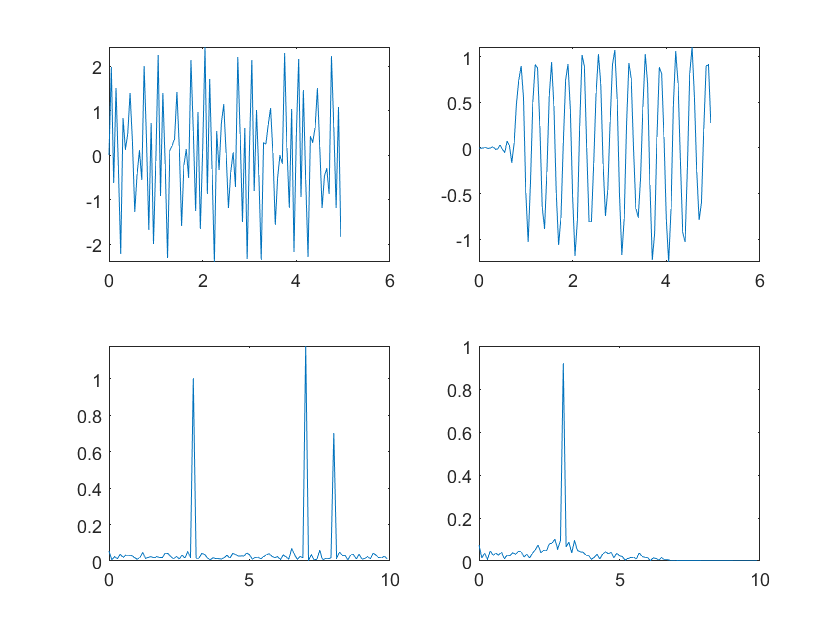1.输出有延迟，FIR滤波器的特性，阶数越大延迟越高，延迟为（N-1)/2,
2.窗函数法不可避免带来一定的频谱泄露，所以输出在3hz幅度变小，附近的频率分量增大
要有基本的数信理论才能看懂，最重要的是两点性质
1.观测时长为T0，则频率分辨率为F0=1/T0
2.采样频率为fs，则最大观测频率为fs
这样才能将坐标系对应上
matlab的滤波器设计如下，需要将滤波器系数导出这样方便使用，有了系数就可以用C语言去实现了。对于入门来说，用什么窗，阶数是多少就随便了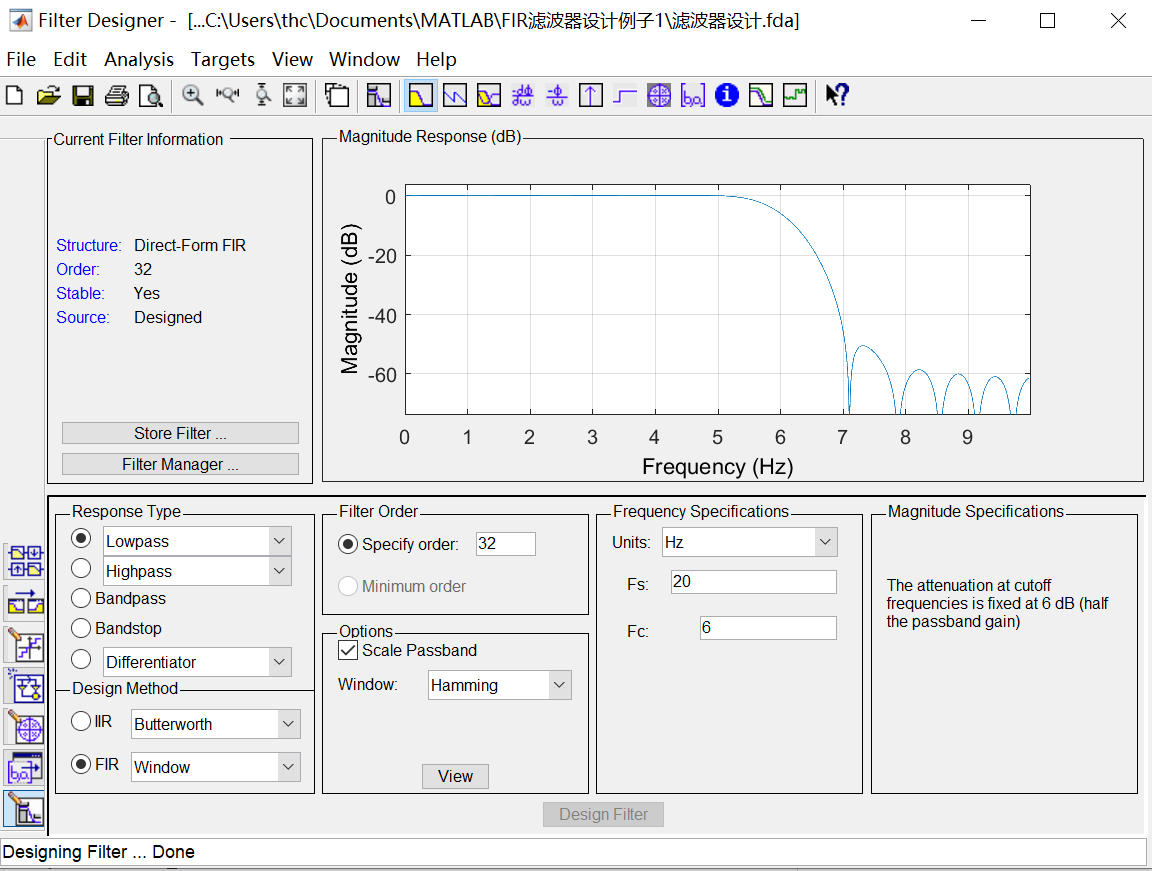展开全文• 充分利用有限冲击响应数字滤波器（Finite Impulse Response digital filter ，FIR）系数的对称特性，借助于MATLAB语言和现场可编程门阵列（FPGA）实现了一种高效的低通滤波器。设计过程中通过简化的VHDL语言编写程序...
• 简述用MATLAB设计低通滤波器的程序，主要针对MATLAB得的初学者!
• %用窗函数法设计线性相位低通滤波器 clear all; wp=0.5*pi; ws=0.6*pi; wd=ws-wp; %hanning窗 N_hann=ceil(8*pi/wd; wc=(wp+ws)/2; if mod(N_hann,2)==0 N_hann=N_hann+1; end hann_win=hanning(N_hann; b_hann=fir1...
• matlab中用汉明窗设计低通滤波器，主要用于心电信号的滤波，可通过直接调节参数进行截止频率的修改。方便好用
• 1 绪 论 1.1 引言 滤波技术是信号分析信号处理技术中的重要分支无论是信号的获取传输还是信号的处理转换都离不开滤波技术滤波技术对信号安全可靠和有效灵活的传递至关重要1 在电子系统中由于滤波器的好坏直接影响...
• Matlab中自带工具箱FDATool也能很方便快速的实现滤波器系数h的整定，可以方便应用到嵌入式（大多数基于FIR滤波设计）、FPGA、DSP里的FIR系数当中，本文将描述基于MATLAB下FDATool生成FIR的实用方法。FDATool打开，...
Matlab中自带工具箱FDATool也能很方便快速的实现滤波器系数h的整定，可以方便应用到嵌入式（大多数基于FIR滤波设计）、FPGA、DSP里的FIR系数当中，本文将描述基于MATLAB下FDATool生成FIR的实用方法。FDATool打开，可以直接在命令窗口键入fdatool回车即可启动，也可以通过UI界面打开，操作如下：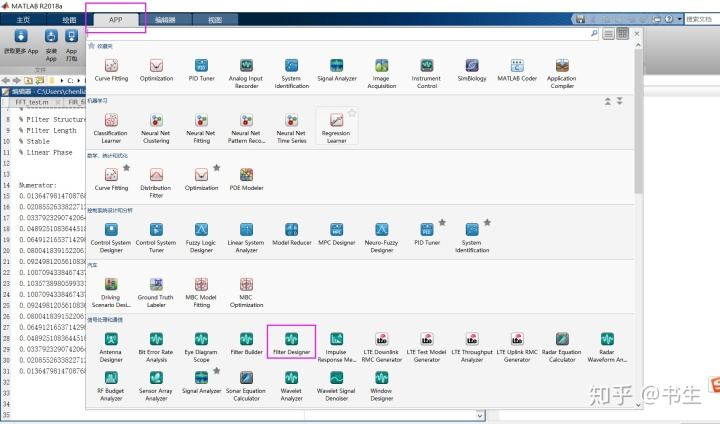UI界面下打开 FDATool这里通过图解快速进行一个FIR设计一个低通滤波器，滤波类型（Filter Type）= Lowpass2. 采样率（Fs） = 1000HZ3. 滤波阶数（order） = 16（阶数越大，滤波品质越好，但相对滞后越大）4. 密度因子（ Density Factor）= order+1 = 175. Fpass（通频带）= 95HZ6. Fstop（截止带）= 105HZ7. Wpass（通频带宽增益期望）= 1DB8. Wstop（截止带宽增益期望）= -40DB具体FDA设计图：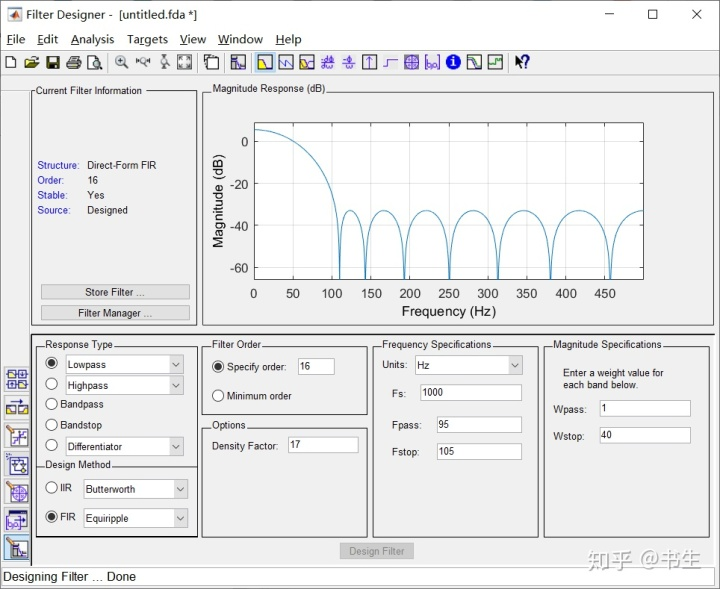FDA设计FIR滤波器生成FIR系数，笔者建议，直接生成ASCII复制出来放到需要运行的工程。File→Export然后保存ASCII格式，这时候MATLAB将会自动打开该文件（也可以通过记事本打开）。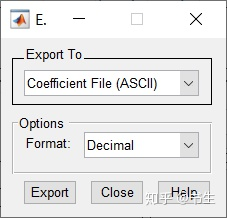FIR系数保存成ACSII格式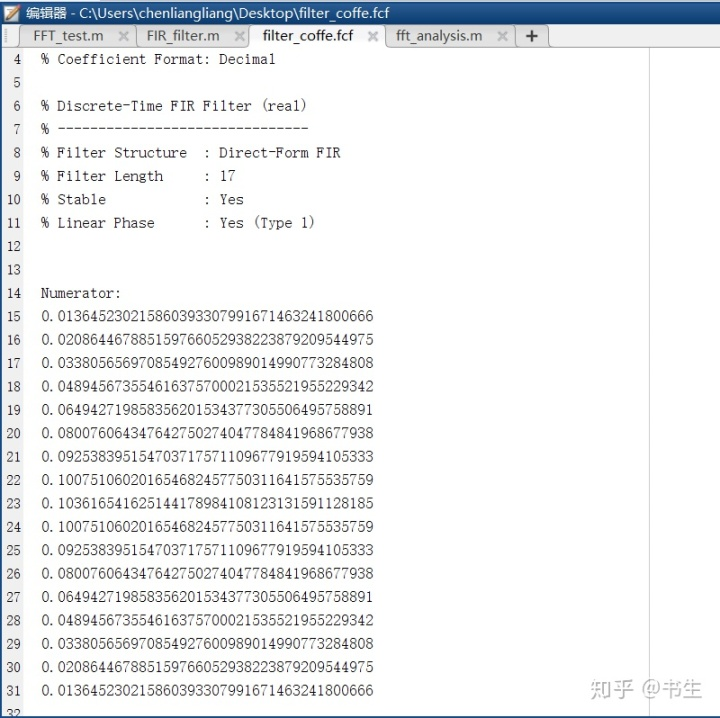FIR系数内容，取Numberator部分最后，用该系数进行FIR滤波处理，详见代码（强烈建议将FIR的h系数拷贝到工程里面）：clear all
clc

%FIR h 1000HZ lopass Fpass = 95HZ  Fstop = 105HZ
h = [0.013645230215860393307991671463241800666
0.020864467885159766052938223879209544975
0.033805656970854927600989014990773284808
0.048945673554616375700021535521955229342
0.064942719858356201534377305506495758891
0.080076064347642750274047784841968677938
0.092538395154703717571109677919594105333
0.100751060201654682457750311641575535759
0.103616541625144178984108123131591128185
0.100751060201654682457750311641575535759
0.092538395154703717571109677919594105333
0.080076064347642750274047784841968677938
0.064942719858356201534377305506495758891
0.048945673554616375700021535521955229342
0.033805656970854927600989014990773284808
0.020864467885159766052938223879209544975
0.013645230215860393307991671463241800666];

Fs = 1000;            % Sampling frequency HZ
T = 1/Fs;             % Sampling period  S
N = 2000;             % Number of signal
t = (0:N-1)*T;        % Time vector

%构造一个信号，其中包含幅值为 0.7 的 50 Hz 正弦量和幅值为 1 的 120 Hz 正弦量
y1 = 0.7*sin(2*pi*50*t) + sin(2*pi*120*t);
%利用h系数进行FIR滤波
y2 = filter(h',1,y1);

%波形快速傅里叶运算
Y1 = fft(y1);
Y2 = fft(y2);

%计算双侧频谱P2
P1_2 = abs(Y1/N);
%取出前面一半进行分析
P1_1 = P1_2(1:N/2+1);
%最终转化为单侧幅频
P1_1(2:end-1) = 2*P1_1(2:end-1);

%计算双侧频谱P2
P2_2 = abs(Y2/N);
%取出前面一半进行分析
P2_1 = P2_2(1:N/2+1);
%最终转化为单侧幅频
P2_1(2:end-1) = 2*P2_1(2:end-1);

%确定频域映射，转化为HZ
f = Fs*(0:(N/2))/N;

subplot(4,1,1);
plot(t,y1);
title('原始信号0.7幅值50HZ，1幅值120HZ')
subplot(4,1,2);
plot(f,P1_1);
title('原始信号FFT分析')
subplot(4,1,3);
plot(t,y2);
title('FIR滤波后波形')
subplot(4,1,4);
plot(f,P2_1);
title('FIR滤波后FFT')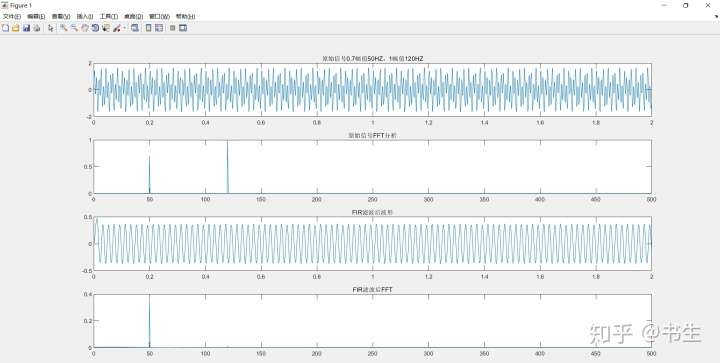FIR数据滤波后图形演示`
展开全文• （一） 设计一个低通滤波器  采样频率 Fs = 50Hz Fpass = 10Hz Fstop = 20Hz 打开matlab，键入fdatool后，会弹出该工具的对话框。然后按照下图设置相关参数，参数列表中的Fs应该是采样频率，Fc是截止频率，即...
• 匿名用户1级2011-05-20 回答%所希望的频率响应在0~0.25*pi之间为1，...%首先需要理解实际的滤波器是没有办法做到理想状态的。如果你要理想状态的话，%就直接就是矩形窗序列乘序列就OK， 矩形窗只能达到20.9dB 的衰减...
• 按照所通过信号频率的不同分为：低通滤波器-允许信号中的低频或直流分量通过，抑制高频分量或干扰和噪声；高通滤波器-允许信号中的高频分量通过，抑制低频或直流分量；带通滤波器-允许一定频段的信号通过，抑制低于...
• 熟悉利用MATLAB软件进行FIR数字滤波器设计，以及对所设计的滤波器进行分析；了解FIR滤波器可实现严格线性相位的条件和特点；熟悉FIR数字滤波器窗函数设计法的MATLAB设计，并了解利用窗函数法设...
• 基于MATLABFIR数字低通滤波器分析和设计
• 连续时间信号以采样频率进行理想采样时通常在采样器前加入一个保护性的前置低通滤波器，该滤波器称为( B )Chap1 P16A.FIR数字滤波器 B.防混叠滤波器 C.IIR数字滤波器 D. 线性相位滤波器16. 对于离散时间系统，从......

# fir低通滤波器matlabmatlab 订阅